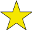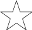## How Much for a Turkey?

Difficulty:(explanation of difficulty)

The following problem is a bit of a classic. It appears in Polya's How to Solve It, but it predates that work.

Among Grandfather's papers a bill was found:

72 turkeys \$_67.9_

The first and last digit of the number that obviously represented the total price of the turkeys have become illegible and are replaced above by blanks. Assuming that each turkey cost the same amount and that amount is an integer number of cents, what was the price of one turkey?
\$

Since each turkey costs an integer number of cents, _679_ must be divisible by 72. If a number is divisible by 72 it must be divisible by both 8 and 9. One can test for divisibility by 8 by checking whether one of the two following conditions holds:

1. The hundreds digit is even and the last two digits are a multiple of 8.
2. The hundreds digit is odd and the last two digits are a multiple of 4 but not 8.

Since the hundreds digit is odd, the last two digits must be a multiple of 4 but not 8. The only number to put in the second blank to make this true would be 2. We now have _6792.

Now, the number _6792 must be divisible by 9, so its digits must add up to a multiple of 9. To accomplish this, the first blank must be 3. Therefore, the 72 turkeys cost \$367.92 and each turkey cost \$5.11.